Adding the voltages by batteries in series

 Why you add the voltages by batteries in series?   You could explain this with the water analogy:    (see wikipedia.org/wiki/Hydraulic_analogy) If you place two pumps in series, then one pump pumps the water , for example, 1 meter higher and another pump in series pumps it again 1 meter higher. In total you pump the water than 2 meters higher.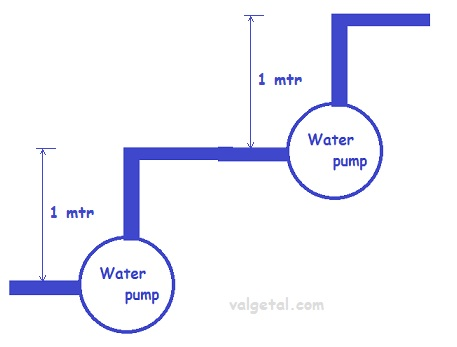A battery is a kind of electron pump. If you place, for example, two batteries of 1 Volt in series, then one battery pumps the electrons 1Volt higher and the other one again 1 Volt higher. The total voltage difference becomes then 2 Volt.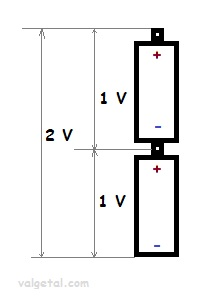But connecting the plus pole of one battery with the minus pole of the other one.... Is this possible? Won't you get some kind of short circuit? What happens then? The following isn't a perfect scientific explanation, but just an attempt to understand the process a little bit... Let's take as an example a Zinc Copper Galvanic Cell  and see what happens when you connect two in series. First we place a zinc electrode in a container with a solution of ZnSO4. An amount of Zn atoms will dissolve from the electrode into the solution, until the created electric potential difference between the Zn electrode and the solution is that high that this process stops. Any Zn atom that likes to dissolve then, is stopped by the force exerted by the negative electrons left in the electrode and by the extra Zn+2 ions in the solution. There is equilibrium when the voltage difference between the electrode and the solution is - 0,76 V (this is just a property of zinc).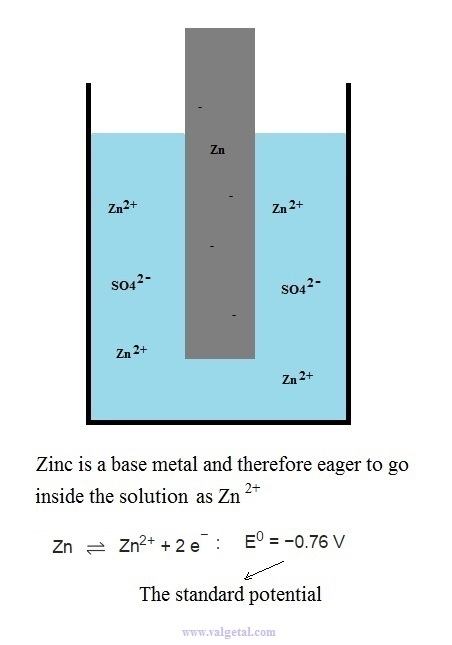I've tried to illustrate this in the following cartoon: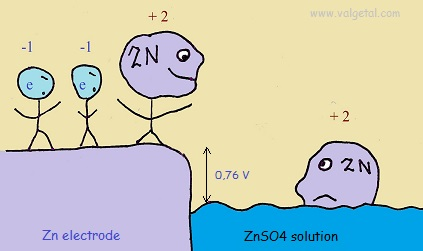The Zinc atoms like to dissolve into the solution.  But when there are already many inside the solution, any new atoms that like to dissolve are stopped by the negative charge of the electrons left in the electrode and the positive charge of the zinc atoms already in the solution. The potential difference will not become greater than - 0,76 V.

 Now we place a copper electrode in another container with a solution of CuSO4. An amount of copper ions will leave the solution and plate (connect) on the electrode, until the created electric potential difference between the copper electrode and the solution is that high that this process stops. When equilibrium is reached, the potential difference (voltage) between the copper electrode and the solution will be + 0,34 V. This is just a property of copper.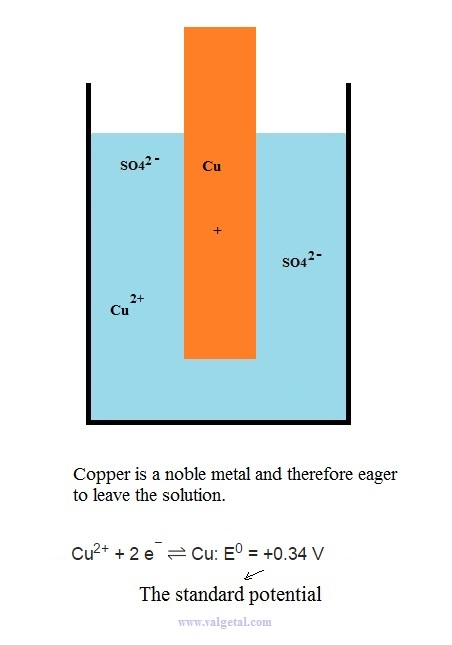Again a cartoon: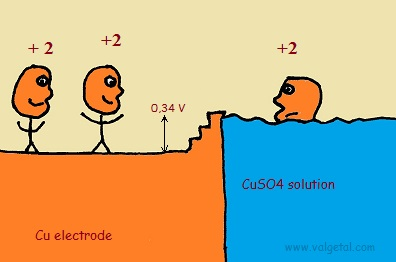The copper ions like to leave the solution and to plate (connect) on the electrode. Although they liberate energy by doing so, there will be a moment that the positively charged copper atoms already in the electrode and the negatively charged SO4 ions in the solution impede that anymore copper ions leave the solution. The potential difference will not become greater than + 0,34 V. (note that the electric potential in the electrode will be higher than the one of the solution, although in the drawing it looks like if it would be lower. This is just to make a similarity with the real world)
 Now we connect in series two galvanic cells. Through the salt bridges SO42- ions can flow (or some other type of ions).  The middle Cu and Zn electrodes are connected by a conducting wire.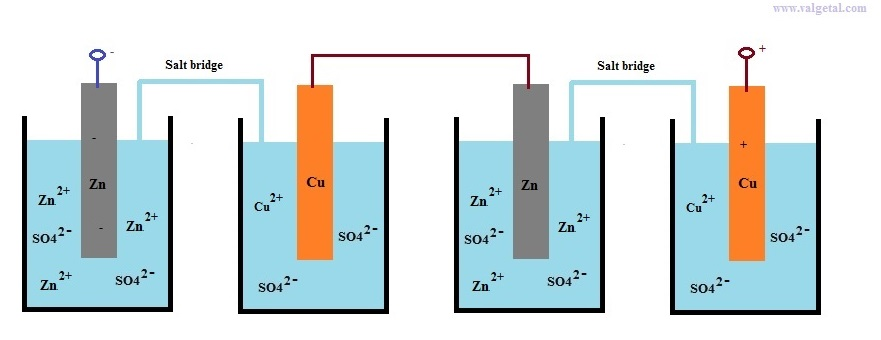Everything is in equilibrium. What has happened with the Zn electrode in the third container when we connected it to the copper electrode? A cartoon to clarify it: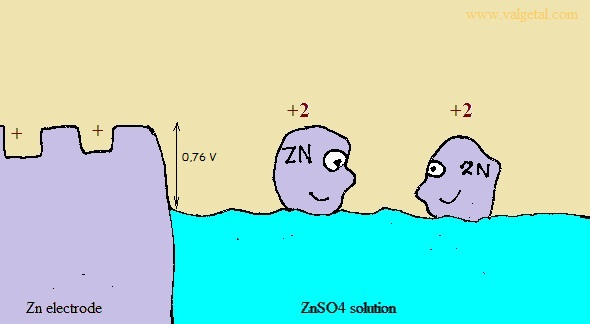Some electrons will have flowed to the Cu electrode with which it is connected. This will cause extra positive charge in the Zn electrode, which will cause in turn that extra Zn atoms will dissolve into the solution, until the potential difference is again 0,76 V and equilibrium has been reached.   It does not matter what is the absolute potential of the zinc electrode or the copper electrode. If you connect an electrode to something and you changes its absolute potential (compared to the earth), then just an amount of zinc and/or copper atoms will leave and/or enter into the solution until equilibrium has been reached again and the standard potential differences are again -0,76 V for the zinc electrode and +0,34 V  for the copper electrode. That the absolute potentials are not important, but yes the potential diffence, you could understand perhaps with the following comparison: image that one person is pulling at your left arm, and two persons are pulling at your right arm (everybody has the same strenght). And then that 100 persons are pulling at your left arm and 101 persons are pulling at your right arm. The net result will be the same (provided that your arms are strong enough...).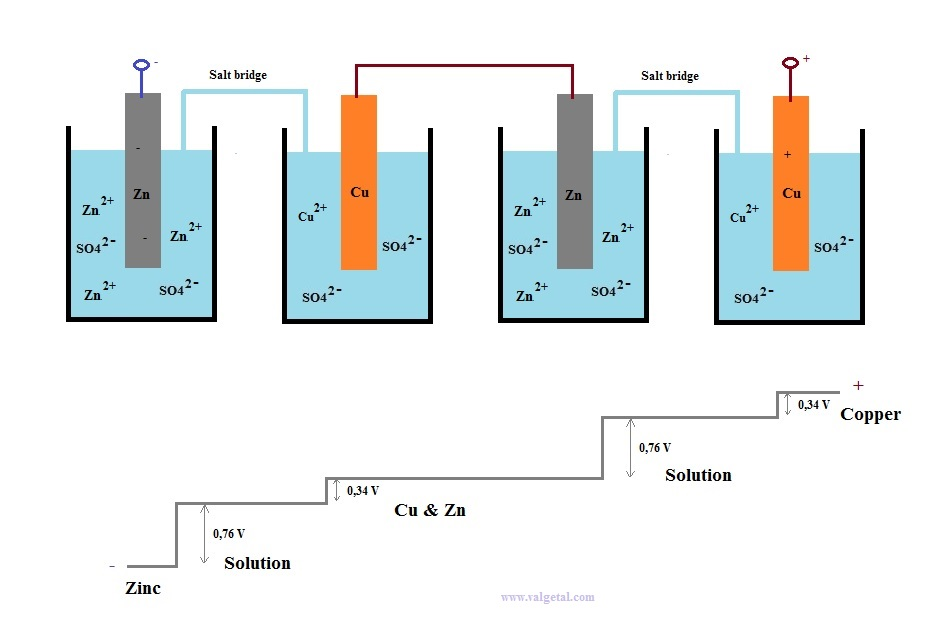Now imagine we are a small positivily charged particle q and we want to travel from the left minus pole
to the right plus pole of the two galvanic cells in series.

First we leave the Zn electrode and enter into the solution. To do this  we have to force
ourselves against the potential difference of 0,76 V (like climbing a hill).

Inside the solution the potential is everywhere the same. We will travel through the salt bridge to
the second container, which will cost us no labour (energy).

Then we go inside the Cu electrode, which will cost us again energy to battle the potential difference of
0,34 V.

Travelling to the Zn electrode costs no energy, because the potential is everywhere the same.

Then we leave the Zn electrode and go inside the solution of the third container. This will cost us energy.

Now we travel through the solution and the salt bridge to the fourth container. This will cost us no energy.

The go inside the Cu electrode will take again some energy.

The total energy (labour) that we have to do will be (0,76 + 0,34 + 0,76 + 0,34) x q = about 2 Volt x q
(q is our charge).

This explanation isn´t perfect, but I hope it´s now understandable that when you place batteries in series,
you have to add the voltages.

By Rinze Joustra,  6 March 2015   www.valgetal.com

A new way of easy nuclear fusion:  www.sem-fusor.com